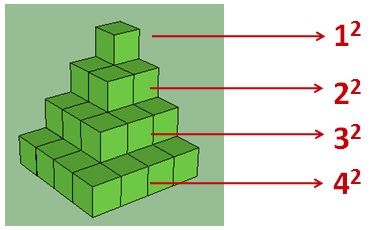# Same Remainder Every TimeIf $n$ is a positive integer, what is the remainder when $\large n \times (n+1) \times (2n+1)$ is divided by 6?

×